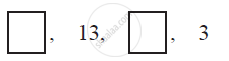# In the following APs find the missing term in the boxes - Mathematics

In the following APs find the missing term in the boxes#### Solution

For this A.P.,

a2 = 13 and

a4 = 3

We know that, an = a + (n − 1) d

a2 = a + (2 − 1) d

13 = a + d (I)

a4 = a + (4 − 1) d

3 = a + 3d (II)

On subtracting (I) from (II), we obtain

−10 = 2d

d = −5

From equation (I), we obtain

13 = a + (−5)

a = 18

a3 = 18 + (3 − 1) (−5)

= 18 + 2 (−5) = 18 − 10 = 8

Therefore, the missing terms are 18 and 8 respectively.

Concept: nth Term of an AP
Is there an error in this question or solution?
Chapter 5: Arithmetic Progressions - Exercise 5.2 [Page 106]

#### APPEARS IN

NCERT Class 10 Maths
Chapter 5 Arithmetic Progressions
Exercise 5.2 | Q 3.2 | Page 106
Share